Question

A projectile is fired with an initial speed of 62.2 m/s at an angle of 43.4 ∘ above the horizontal on a long flat firing range.Determine the direction of the projectile 1.32 s after firing.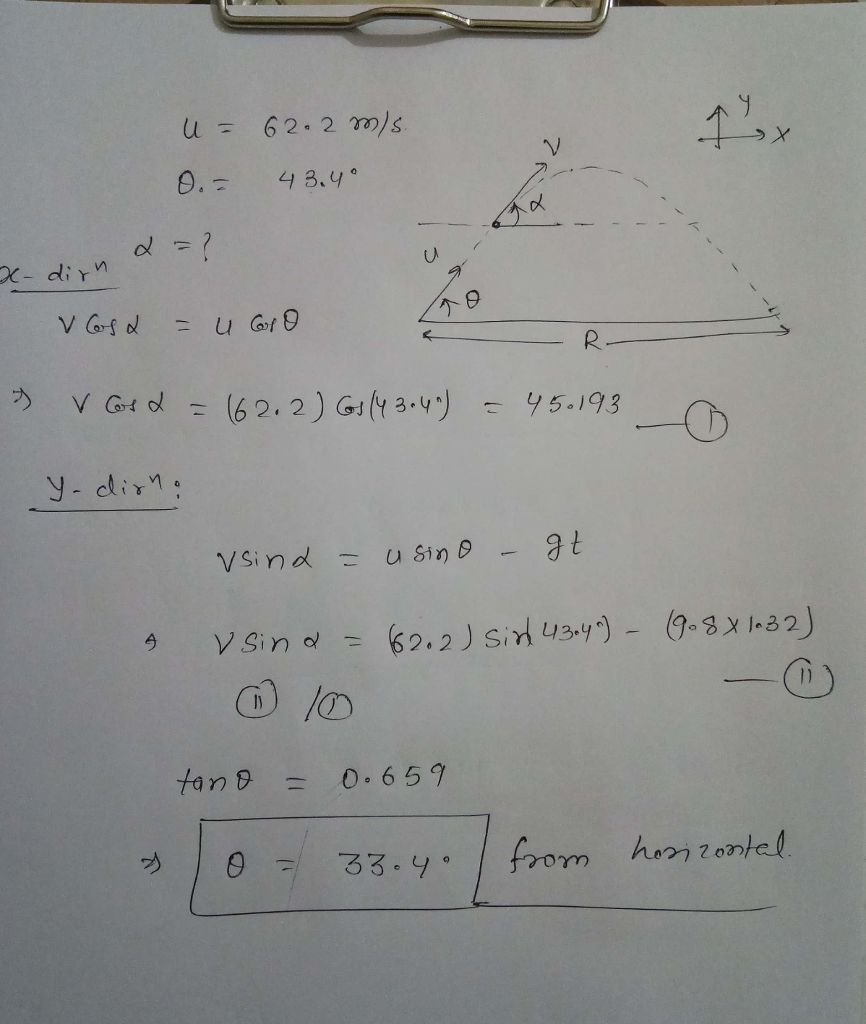#### Earn Coins

Coins can be redeemed for fabulous gifts.

Similar Homework Help Questions
• ### A projectile is fired with an initial speed of 20. m/s at an angle of 42.2°...

A projectile is fired with an initial speed of 20. m/s at an angle of 42.2° above the horizontal on a long flat firing range. Determine, a) the maximum height reached by the projectile, b) the total time in the air, c) the total horizontal distance covered (that is, the range), d) the speed of the projectile 1.50 s after firing. e) direction of the speed at 1.50 s.

• ### A projectile is fired with an initial speed of 36.2m/s at an angle of 41.1 degrees...

A projectile is fired with an initial speed of 36.2m/s at an angle of 41.1 degrees above the horizontal on a long flat firing range. determine maxiumum height reached by projectile total time in the air determine the range speed of the projectile 1.80s after firing

• ### Determine the total time in the ar Constants A projectile is fired with an initial speed...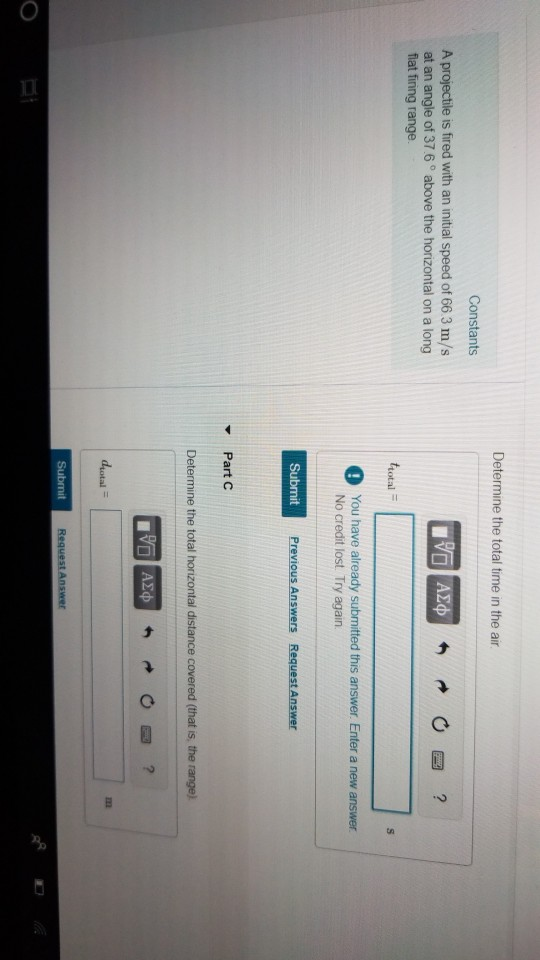Determine the total time in the ar Constants A projectile is fired with an initial speed of 66 3 m/s at an angle of 37 6 ° above the horizontal on a long total O You have already submitted this answer. Enter a new answer No credit lost. Try again Submit ▼ Part C Determine the total horizontal distance covered (that is, the range) dyotal Constants Part D A projectile is fired with an initial speed of 66.3 m/s at...

• ### A projectile is fired with an initial speed of 37.4 m/s at an angle of 45.0...

A projectile is fired with an initial speed of 37.4 m/s at an angle of 45.0 ° above the horizontal on a long flat firing range.Part ADetermine the maximum height reached by the projectile. Express your answer using three significant figures and include the appropriate units. Part B Determine the total time in the air Part C Determine the total horizontal distance covered (that is, the range).

• ### ASSIGNMENT PROBLEMS 1: A projectile is fired with an initial speed of 60.0 m/s at an...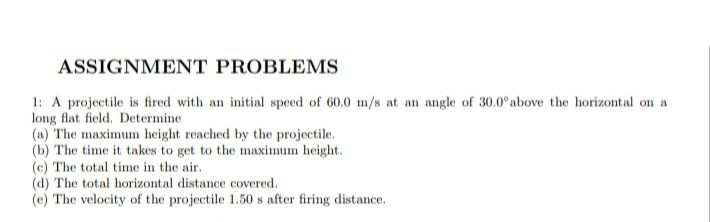ASSIGNMENT PROBLEMS 1: A projectile is fired with an initial speed of 60.0 m/s at an angle of 30.0°above the horizontal on a long flat field. Determine (a) The maximum height reached by the projectile. (b) The time it takes to get to the maximum height. (c) The total time in the air. (d) The total horizontal distance covered. (e) The velocity of the projectile 1.50 s after firing distance.

• ### A projectile is fired with an initial speed of 37.8 m/s at an angle of 41...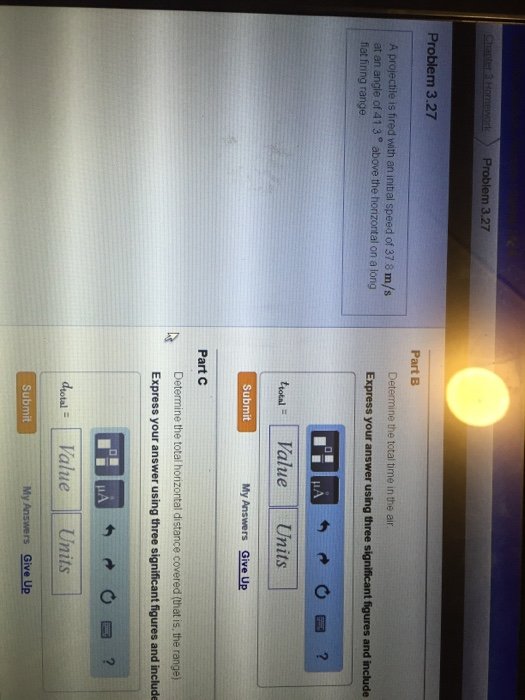A projectile is fired with an initial speed of 37.8 m/s at an angle of 41 3 degree above the horizontal on a long flat fining range

• ### A projectile is fired with Aprojectile is fired with an initial speed of 37.9 m/s at...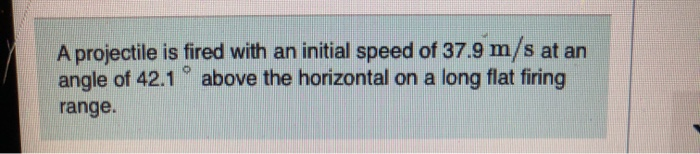A projectile is fired with Aprojectile is fired with an initial speed of 37.9 m/s at an angle of 42.1 above the horizontal on a long flat firing range. Part B Determine the total time in the air. Express your answer using three significant figures and include the appropriate units. HA ? trotal 5.081 Submit Previous Answers Request Answer xIncorrect; Try Again; 8 attempts remaining Review your calculations; you may have made a rounding error or used the wrong number...

• ### A projectile is fired with initial velocity v0 = 95 m/s at an angle θ = 50°.

Problem 8:A projectile is fired with initial velocity v0 = 95 m/s at an angle θ = 50°. After 5 s it strikes the top of a hill. What is the elevation of the hill above the point of firing? At what horizontal distance from the gun does the projectile land?

• ### a projectile is fired with an initial speed of 2000 m/s at an angle 21 degrees...

a projectile is fired with an initial speed of 2000 m/s at an angle 21 degrees above the horizontal. if air resistance is neglected , what is the velocity in m/s at the highest point of trajectory ?